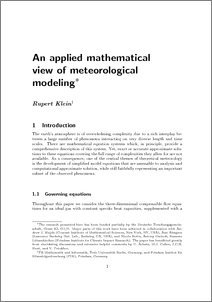Repository: Freie Universität Berlin, Math Department

# An applied mathematical view of meteorological modeling

Klein, R. (2004) An applied mathematical view of meteorological modeling. Applied Mathematics Entering the 21st century; Invited talks from the ICIAM 2003 Congress., 116 . pp. 177-219.Preview

2MB

## Abstract

The earth’s atmosphere is of overwhelming complexity due to a rich interplay between a large number of phenomena interacting on very diverse length and time scales. There are mathematical equation systems which, in principle, provide a comprehensive description of this system. Yet, exact or accurate approximate solutions to these equations covering the full range of complexities they allow for are not available. As a consequence, one of the central themes of theoretical meteorology is the development of simplified model equations that are amenable to analysis and computational approximate solution, while still faithfully representing an important subset of the observed phenomena.

Item Type: Article Mathematical and Computer Sciences > Mathematics Department of Mathematics and Computer Science > Institute of Mathematics > Geophysical Fluid Dynamics Group 472 Ulrike Eickers 24 Jun 2009 13:46 03 Mar 2017 14:40

Repository Staff Only: item control page